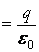# Short Notes: Electric Charges and Fields – Part 2# Principle of SuperpositionIf a system contains a number of interacting charges, then the force on a given charge is equal to the vector sum of forces exerted on this charge by all remaining charges. This is called the principle of superposition. Suppose a system has charges ε, at positions# Electric Field Strength

The region in which a charged particle experiences a force is called the electric field and the electric field strength at any point is the electric force experienced by per unit infinitesimal positive charge when placed at that point.

Asis infinitesimal, so above equation is best represented asSI Unit: The SI unit of force is Newton (N) and SI unit of charge is coulomb; so SI unit of electric field strength is Newton/coulomb abbreviated as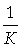Electric field strength due to a point charge: Let P be a point at a distance r from point charge +q. If a test charge(positive and infinitesimal) is placed at point P, then electric force at chargeis.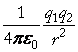q1q2

Thus, the magnitude of electric field strength isand its direction is away from charge +q. And if point charge is negative, then the electric field strength has same magnitudebut its direction is towards point charge (– q).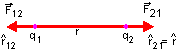Electric field strength due to a system of charges : If a system contains charges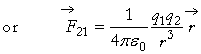at positions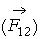respectively from origin O, andrespectively are the electric field strengths at point P having position vector, then electric field strength at P is the vector sum ofi.e.,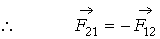# Electric Field Line

An electric field line is a path of a positively charged particle in an electric field when it is initially at rest.

Characteristics:

(i) The electric field lines appear to start from positive charge and terminate at negative charge. And if there is a single charge, they may start or end at infinity.

(ii) The tangent drawn at any point on the field line gives the direction of electric field strength at that point and the direction of force acting on a positive charge at that point.

(iii) No two electric field lines can intersect each other because if they do so, then there can be two tangents at the point of intersection; which would mean two directions of resultant electric field strength at one point, which is impossible. Therefore, intersection of two electric field lines is not possible.

(iv) The electric field lines have tendency to contract lengthwise like a stretched elastic string and separate from each other laterally. It is so because opposite charges attract and similar charges repel.

(v) The electric filed lines do not form any closed loops.

(vi) The equidistant electric lines of force represent uniform electric field while electric lines of forces at different separations represent non-uniform electric field (figure).

(vii) Number of electric field lines passing unit area surrounding that point is electric intensity at that point.

(viii) Electric field lines originate normally to the surface of charged body.

# Electric Dipole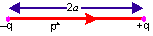A system containing two equal and opposite charges separated by a small distance is called an electric dipole. Dipole moment of electric dipole having charges at separation 2a is.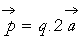Unit of dipole moment is coulomb metre.

It is a vector quantity, with the direction from –q to +q.

Electric field at an axial point P of a dipole: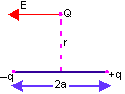along the line joining – q to + q.

Electric field at an equatorial point Q of a dipole:parallel to line joining + q to – q.

# Torque on a dipole in a uniform electric field

Consider an electric dipole placed in an uniform electric field of strength E in such a way that its dipole moment q to qmakes an anglewith the direction of. The charges of dipole are –q and +q at separation 2a. The dipole moment of electric dipole is,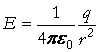Force: The force on charge + q is,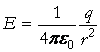, along direction of fieldThe force on charge – q is,, opposite to direction of fieldObviously forces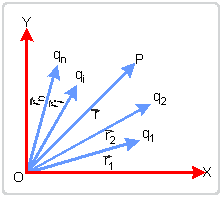and q1,q2,…….qn      are equal in magnitude but opposite in direction; hence net force on electric dipole in uniform electric field is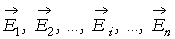As net force on electric dipole is zero, so dipole does not undergo any translatory motion.

Torque: The forcesand q1,q2,…….qn  form a couple (or torque) which tends to rotate and align the dipole along the direction of electric field. This couple is called the torque and is denoted by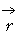.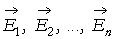Clearly, the magnitude of torque depends on orientationof the electric dipole relative to electric field. Torqueis a vector quantity whose direction is perpendicular to both q to q and.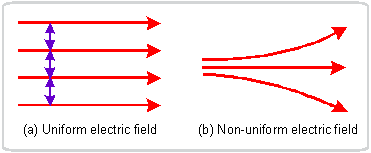Question: Define electric dipole moment in terms of torque acting on it when placed in an uniform electric field.

Ans. Torque on an electric dipole of moment p when placed in a uniform electric field E isThat is the electric dipole moment is numerically equal to the torque acting on it when it is placed in a uniform electric field of strength 1 NCwith its dipole moment perpendicular to the direction of electric field.

# Electric Flux and Gauss`s Theorem

The electric flux through a surface is the total number of electric field lines of force diverging normally from that surface.

Electric flux through surface element  θ = 90° is Φ = E × πr2 90°, where θ = 0° is electric field strength,

and electric flux through entire closed surface is Φ = E × πr2 cos0° =Eπr2

Gauss’s Theorem: It states that the net electric flux diverging normally from a closed surface is equal totimes the net charge enclosed by the surface, i.e.,